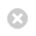Close

UCL Centre for Languages & International Education (CLIE)

Home# Mathematics (UPCSE)

Find out more about the Mathematics foundation subject on the UPCSE. Learn about what you will study, teaching methods, assessments and recommended reading.

## Key information

Subject title: Mathematics

Subject type: optional

UPC Pathway: UPCSE

Subject leader: Dr Ramesh Satkurunath

Number of students (2022-23): 59

## Subject description

The Mathematics subject is an optional part of the Undergraduate Preparatory Certificate for Science and Engineering (UPCSE) course. We’ll provide you with an in-depth introduction into Pure Maths. You’ll develop your skills in Algebra, Trigonometry, Geometry and their related topics. The subject aims to prepare students thoroughly with the skills needed to study at UK universities. Students typically go on to study degrees such as Mathematics, Engineering, Life Sciences and Physical Sciences.

The syllabus covers the following topics:

### Algebra

• Long division
• Factorisation
• Fundamental theorem of algebra
• Remainder and factor theorem
• Partial fractions
• Proof by induction
• Binomial theorem and generalised binomial theorem

### Differentiation

• Limits at a point
• Differentiation from first principles
• Rules of differentiation (product rule, quotient rule, chain rule)
• Implicit differentiation
• Tangents and normals
• Finding and classifying stationary points
• Optimisation

### Curve Sketching

• x-intercepts, y-intercepts, points of inflection, end behaviour/limits at infinity, vertical asymptotes
• Numerical methods, Newton-Raphson method, bisection method
• Transformations of curves

### Integration

• Indefinite integrals
• Integration by parts, integration by substitution
• Definite integration
• Trigonometric substitutions
• Using integration to find area and volumes of revolution

### Series

• Arithmetic and geometric progressions/series
• Method of differences/telescoping series
• Sum of squares and cubes
• Maclaurin Series, Taylor Series

### Complex numbers

• Complex arithmetic, properties of complex numbers
• Factorisation of polynomials over complex numbers
• Argand diagram
• Polar form, exponential form
• De Moivre’s theorem
• Loci
• Transformation of the complex plane

### Vectors

• Vector arithmetic
• Scalar product, cross product, triple product
• Equations of lines and planes
• Finding the angle/distance between planes/lines/points
• Finding the intersection of lines/planes.

## How we teach Mathematics

You'll have two online lectures every week. Both lectures will be available as recordings. You’ll also have two in-person smaller-group tutorials. During the tutorials, you’ll work on Pure Maths exercises based on the lectures. We expect you to carry out independent study and problem-solving outside of your classes.

Office hours will be available for additional support throughout your foundation year.

## Intended learning outcomes

By the end of the subject, you should be able to:

• state and prove the main theoretical results the syllabus is based on
• apply the theory to problem-solving
• present well-written solutions to problems with good attention to detail and a logical structure
• work independently on mathematics exercises, taking responsibility for your own understanding and asking for help when necessary
• confidently discuss mathematical ideas in English, using correct vocabulary.

## Overview of Summative Assessment

TermAssessmentWeighting
OneEnd of Term Written Exam (2 hours)10%
TermEnd of Term Written Exam (2 hours)10%
ThreeFinal Written Exam (3 hours)60%
Throughout the yearClass Tests (1 hour)20%
100% Total

Assessment weighting is for the 2021/22 academic year. This may change for 2022/23 entry.

Before starting the course, you should prepare by revising high school work. You’ll need to be comfortable with exponentials, logarithms and have strong trigonometry knowledge.

You should be able to work with all six trigonometric functions (sine, cosine, tangent, cotangent, secant, cosecant) and the inverse trigonometric functions. You should also be familiar with standard trigonometric identities and using them in proofs. Any standard high school mathematics text should cover this Pure Maths material (e.g., ‘Mathematics- The Core Course for A-level’ – see below).